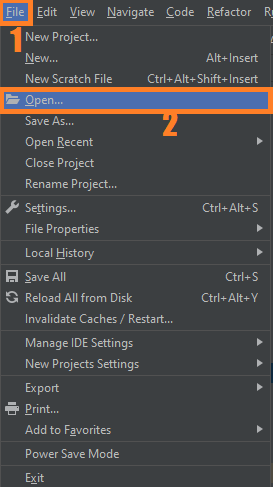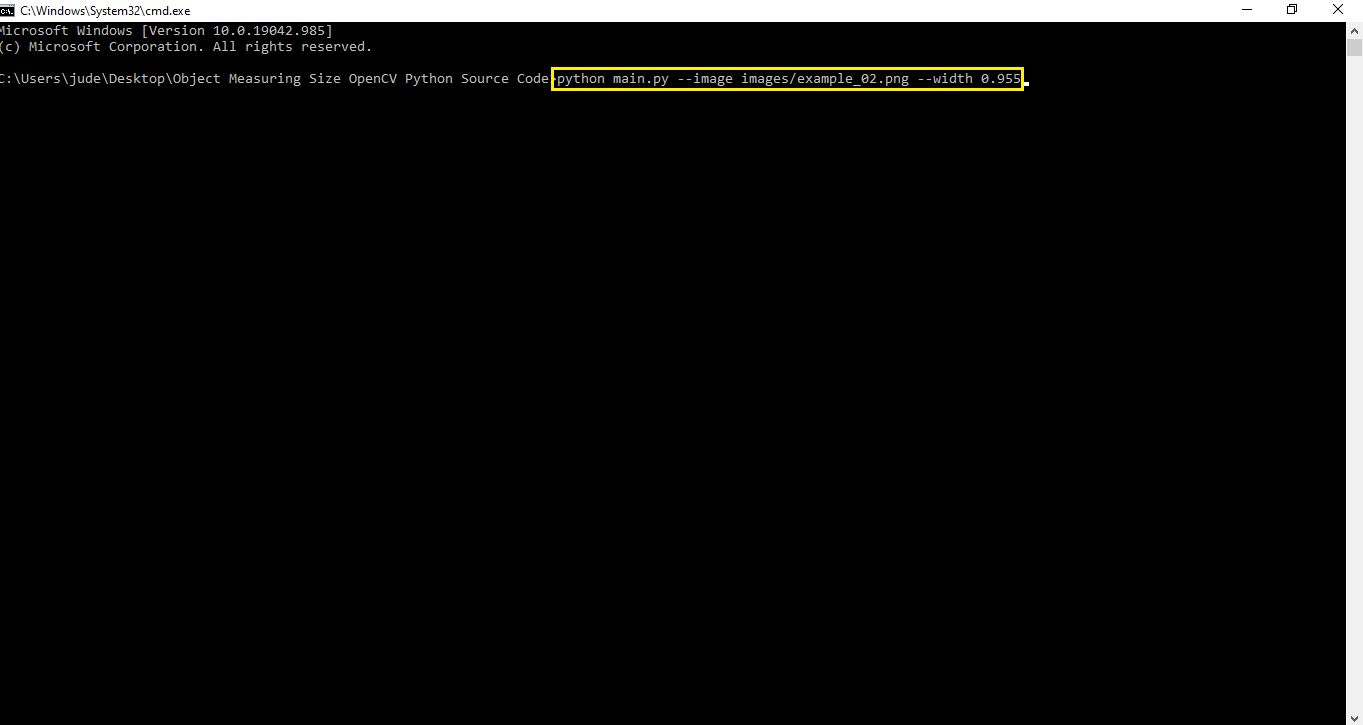# Object Measuring Size OpenCV Python With Source Code

## Object Measuring Size OpenCV Python With Source Code

The Object Measuring Size OpenCV Python was developed using Python OpenCV, The project provides a script to read an image and based on the dimensions of a reference object find the dimensions of other objects in a scene.

A Object Measuring we will learn how to perform object measurement using OpenCV and Python. We will use an image as our guide and find the measurement size of objects placed in this region.

By the way if you are new to python programming and you don’t know what would be the the Python IDE to use, I have here a list of Best Python IDE for Windows, Linux, Mac OS that will suit for you. I also have here How to Download and Install Latest Version of Python on Windows.

To start executing Object Measuring Size With Source Code, make sure that you have installed Python 3.9 and PyCharm in your computer.

## Object Measuring Size OpenCV Python With Source Code : Steps on how to run the project

Time needed: 5 minutes.

These are the steps on how to run Object Measuring Size OpenCV Python With Source Code

First, download the given source code below and unzip the source code.• Step 2: Import the project to your PyCharm IDE.• Step 3: Run the project.

last, run the project with the command “python main.py –image images/example_02.png –width 0.955”## Installed Libraries

```from scipy.spatial import distance as dist
from imutils import perspective
from imutils import contours
import numpy as np
import argparse
import imutils
import cv2```

## Complete Source Code

```# USAGE
# python object_size.py --image images/example_01.png --width 0.955
# python object_size.py --image images/example_02.png --width 0.955
# python object_size.py --image images/example_03.png --width 3.5

# import the necessary packages
from scipy.spatial import distance as dist
from imutils import perspective
from imutils import contours
import numpy as np
import argparse
import imutils
import cv2

def midpoint(ptA, ptB):
return ((ptA + ptB) * 0.5, (ptA + ptB) * 0.5)

# construct the argument parse and parse the arguments
ap = argparse.ArgumentParser()
help="path to the input image")
help="width of the left-most object in the image (in inches)")
args = vars(ap.parse_args())

# load the image, convert it to grayscale, and blur it slightly
gray = cv2.cvtColor(image, cv2.COLOR_BGR2GRAY)
gray = cv2.GaussianBlur(gray, (7, 7), 0)

# perform edge detection, then perform a dilation + erosion to
# close gaps in between object edges
edged = cv2.Canny(gray, 50, 100)
edged = cv2.dilate(edged, None, iterations=1)
edged = cv2.erode(edged, None, iterations=1)

# find contours in the edge map
cnts = cv2.findContours(edged.copy(), cv2.RETR_EXTERNAL,
cv2.CHAIN_APPROX_SIMPLE)
cnts = imutils.grab_contours(cnts)

# sort the contours from left-to-right and initialize the
# 'pixels per metric' calibration variable
(cnts, _) = contours.sort_contours(cnts)
pixelsPerMetric = None

# loop over the contours individually
for c in cnts:
# if the contour is not sufficiently large, ignore it
if cv2.contourArea(c) < 100:
continue

# compute the rotated bounding box of the contour
orig = image.copy()
box = cv2.minAreaRect(c)
box = cv2.cv.BoxPoints(box) if imutils.is_cv2() else cv2.boxPoints(box)
box = np.array(box, dtype="int")

# order the points in the contour such that they appear
# in top-left, top-right, bottom-right, and bottom-left
# order, then draw the outline of the rotated bounding
# box
box = perspective.order_points(box)
cv2.drawContours(orig, [box.astype("int")], -1, (0, 255, 0), 2)

# loop over the original points and draw them
for (x, y) in box:
cv2.circle(orig, (int(x), int(y)), 5, (0, 0, 255), -1)

# unpack the ordered bounding box, then compute the midpoint
# between the top-left and top-right coordinates, followed by
# the midpoint between bottom-left and bottom-right coordinates
(tl, tr, br, bl) = box
(tltrX, tltrY) = midpoint(tl, tr)
(blbrX, blbrY) = midpoint(bl, br)

# compute the midpoint between the top-left and top-right points,
# followed by the midpoint between the top-righ and bottom-right
(tlblX, tlblY) = midpoint(tl, bl)
(trbrX, trbrY) = midpoint(tr, br)

# draw the midpoints on the image
cv2.circle(orig, (int(tltrX), int(tltrY)), 5, (255, 0, 0), -1)
cv2.circle(orig, (int(blbrX), int(blbrY)), 5, (255, 0, 0), -1)
cv2.circle(orig, (int(tlblX), int(tlblY)), 5, (255, 0, 0), -1)
cv2.circle(orig, (int(trbrX), int(trbrY)), 5, (255, 0, 0), -1)

# draw lines between the midpoints
cv2.line(orig, (int(tltrX), int(tltrY)), (int(blbrX), int(blbrY)),
(255, 0, 255), 2)
cv2.line(orig, (int(tlblX), int(tlblY)), (int(trbrX), int(trbrY)),
(255, 0, 255), 2)

# compute the Euclidean distance between the midpoints
dA = dist.euclidean((tltrX, tltrY), (blbrX, blbrY))
dB = dist.euclidean((tlblX, tlblY), (trbrX, trbrY))

# if the pixels per metric has not been initialized, then
# compute it as the ratio of pixels to supplied metric
# (in this case, inches)
if pixelsPerMetric is None:
pixelsPerMetric = dB / args["width"]

# compute the size of the object
dimA = dA / pixelsPerMetric
dimB = dB / pixelsPerMetric

# draw the object sizes on the image
cv2.putText(orig, "{:.1f}in".format(dimA),
(int(tltrX - 15), int(tltrY - 10)), cv2.FONT_HERSHEY_SIMPLEX,
0.65, (255, 255, 255), 2)
cv2.putText(orig, "{:.1f}in".format(dimB),
(int(trbrX + 10), int(trbrY)), cv2.FONT_HERSHEY_SIMPLEX,
0.65, (255, 255, 255), 2)

# show the output image
cv2.imshow("Image", orig)
cv2.waitKey(0)```